Do you like the article?
Share it with others -
Use new possibilities of MetaTrader 5

# Transferring an Indicator Code into an Expert Advisor Code. Indicator Structure

25 September 2007, 07:40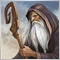4
28 447

### Introduction

For a better understanding the author recommends reading the following material:

1. MetaQuotes Software Corp. Features of Custom Indicators Creation. http://articles.mql4.com/en/articles/1497
2. Nikolay Kositsin. Multiple Null Bar Re-Count in Some Indicators. http://articles.mql4.com/en/articles/1411
Before starting to dwell on the topic of the article, indicated in the title, the following question would be rather appropriate: "What for do we need to transfer an indicator code into an EA code, if in the majority of cases an EA, operating with custom indicators, looks like much easier than its analogue, which contains all necessary for the operation custom indicators inside its own code? Especially if we take into account the fact that if a code is written correctly, the results in both cases will be absolutely identical!"

In my opinion, it is necessary in two cases:

1. If in EA calculation values calculated on a zero bar are not used at all, we naturally would like to omit unnecessary recalculation on the zero bar and on the first bar. This allows shortening thrice the time of such an EA optimization, which is rather relevant in a very complicated and resource-intensive code!
2. Commercial usage of an Expert Advisor with the maximal protection of its code against decompiling.

In the second case the situation is quite clear and the code transferring is quite reasonable. And in the first case in the majority of situations it is much easier to rewrite custom indicators' codes, excluding unnecessary calculations! Naturally, such indicators will be suitable only for Expert Advisors, and not trading! So, let us start our discussion from this variant of the problem solution.

### Example of Indicator Optimization

First of all I would like to draw your attention to the following code fragment of a custom indicator:

```int start()
{
int limit;
int counted_bars = IndicatorCounted();
//---- the last calculated bar will be recalculated
if(counted_bars > 0)
counted_bars--;
limit = Bars - counted_bars - 1;
//---- the main cycle
for(int i = limit; i >= 0; i--)
{
//----
ExtBlueBuffer[i] = iMA(NULL, 0, JawsPeriod, 0, MODE_SMMA,
PRICE_MEDIAN, i);
ExtRedBuffer[i] = iMA(NULL, 0, TeethPeriod, 0, MODE_SMMA,
PRICE_MEDIAN, i);
ExtLimeBuffer[i] = iMA(NULL, 0, LipsPeriod, 0, MODE_SMMA,
PRICE_MEDIAN, i);
}
//----
return(0);
}```

In this case the following line is relevant for us:

```if(counted_bars > 0)
counted_bars--;```

The meaning of this checking with diminution of the variable 'counted_bars' value by one is the following: if a custom indicator does not include this line, it may send wrong values from its buffers into an EA, when the zero bar is changed. The indicator curve in the EA will have quite a "crumpled" form.

In custom indicators, the variables 'limit' and 'counted_bars' may have different names, but the program code must have these checkups! I suppose this explanation is enough to clear up claims of some EA writers, that in MetaTrader data from indicator buffer and the same data received from a custom indicator are not identical. If an indicator code and an EA code are written correctly, no matter how difficult the indicator code is, the data will be always the same!

But it should be noted here, that some smoothing algorithms are sensitive to the reference point, from which the smoothing starts. I.e. in order to have identical values, the numbers of the oldest bars, from which the recalculation of all bars starts, in cycles both in the indicator and in the indicator code inside the EA should coincide.

Here is an example, explaining this method of an indicator code optimization for its quicker operation in an EA. In the main indicator cycle change zero into one, after that the indicator discontinues to recalculate its value on the zero bar.

```// instead of
for(int i = limit; i >= 0; i--)
// write
for(int i = limit; i >= 1; i--)```

As a result the source code will look like the following way:

```int start()
{
int limit;
int counted_bars = IndicatorCounted();
//---- the last bar will be recalculated
if(counted_bars > 0)
counted_bars--;
limit = Bars - counted_bars - 1;

//---- the main cycle //now the cycle ends in 1
for(int i = limit; i >= 1; i--)
{
//----
ExtBlueBuffer[i] = iMA(NULL, 0, JawsPeriod, 0, MODE_SMMA,
PRICE_MEDIAN, i);
ExtRedBuffer[i] = iMA(NULL, 0, TeethPeriod, 0, MODE_SMMA,
PRICE_MEDIAN, i);
ExtLimeBuffer[i] = iMA(NULL, 0, LipsPeriod, 0, MODE_SMMA,
PRICE_MEDIAN, i);
}
//----
return(0);
}```
I will repeat, that the above method is for EAs working only on closed bars, i.e. on all bars except the zero one!

If you are seriously going to use your EA in real trading and trust it your money, you should carefully check all the details in your Expert Advisor, as well as the indicators with which the EA works. Moreover you should do it yourself! I think it is much easier and cleverer to devote a couple of days to a thorough understanding of the indicator structure and methods of optimization of its code, than with patience use for three months an EA that receives values from a roughly written indicator!

So, it must be clear that one needs serious reasons for transferring an indicator code into an EA code. If an indicator is written correctly, the EA's operation will not be much slower without this. It is easier to write first an EA code using custom indicators and to conduct the check-out in this form. And if the EA really shows perfect results, the code can be further optimized, one by one changing calls to custom indicators into indicator code fragments.

And it must be noted that the tested loss and profit values should not be amended after changing the EA code!

The number of existing indicators is very large, each of them has its unique code. That is why one can hardly create a universal method of code transferring for all indicators. The problem is worsened by the fact, that one and the same custom indicator may be represented in an EA code several times. If an indicator code is more or less simple, it can be written into a custom function and changing custom indicators into functions in such a case is quite easy. But very often an EA code achieves such dimensions, that it is almost impossible to detect an error in it. And all our efforts are in vain.

### General Scheme of an Indicator Structure

Before starting to dwell on the main topic of the article, first let us analyze an indicator structure from the point of view of a programmer, who is interested in the indicator as a future part of an EA code:
```//+------------------------------------------------------------------+
//|                                                IndicatorPlan.mq4 |
//|                                        https://www.metaquotes.net/ |
//+------------------------------------------------------------------+
//---- drawing the indicator in the main window
#property indicator_chart_window
//---- number of indicator buffers
#property indicator_buffers 1
//---- indicator color
#property indicator_color1 Gold
//---- INPUT PARAMETERS OF THE INDICATOR
extern int period0 = 15;
extern int period1 = 15;
extern int period2 = 15;
//---- indicator buffers
double Ind_Buffer0[];
double Ind_Buffer1[];
double Ind_Buffer2[];
//+------------------------------------------------------------------+
//| Custom indicator initialization function                         |
//+------------------------------------------------------------------+
int init()
{
//---- defining the graph execution style
SetIndexStyle(0, DRAW_LINE);
//---- 3 indicator buffers are used for calculation
IndicatorBuffers(3);
SetIndexBuffer(0, Ind_Buffer0);
SetIndexBuffer(1, Ind_Buffer1);
SetIndexBuffer(2, Ind_Buffer2);
//---- end of initialization
return(0);
}
//+------------------------------------------------------------------+
//| Custom indicator iteration function                              |
//+------------------------------------------------------------------+
int start()
{
//---- Checking whether the bars number is enough for further calculation
if(Bars < period0 + period1 + period2)
return(0);
//----+ Insertion of variables with a floating point
double Resalt0, Resalt1, Resalt2;
//----+ Insertion of integer variables and getting calculated bars
int limit, MaxBar,bar, counted_bars = IndicatorCounted();
//---- checking for possible errors
if(counted_bars < 0)
return(-1);
//---- the last calculated bar must be recalculated
if(counted_bars > 0)
counted_bars--;
//---- defining the number of the oldest bar,
// starting from which new bars will be recalculated
limit = Bars - counted_bars - 1;
//---- defining the number of the oldest bar,
// starting from which new bars will be recalculated
MaxBar = Bars - 1 - (period0 + period1 + period2);
//---- initialization of zero
if(limit > MaxBar)
{
limit = MaxBar;
for(bar = Bars - 1; bar >= MaxBar; bar--)
{
Ind_Buffer0[bar] = 0.0;
Ind_Buffer1[bar] = 0.0;
Ind_Buffer2[bar] = 0.0;
}
}
//----+ THE FIRST CYCLE OF INDICATOR CALCULATION
for(bar = limit; bar >= 0; bar--)
{
// Here code of the variable Resalt1 calculation based on the external
// variable period1
Ind_Buffer1[bar] = Resalt1;
}
//----+ THE SECOND CYCLE OF INDICATOR CALCULATION
for(bar = limit; bar >= 0; bar--)
{
// Here code of the variable Resalt2 calculation
// based on the values of the buffer Ind_Buffer1[]
// and external variable period2
Ind_Buffer2[bar] = Resalt2;
}
//----+ THE MAIN CYCLE OF INDICATOR CALCULATION
for(bar = limit; bar >= 0; bar--)
{
// Here code of the variable Resalt0 calculation
// based on the values of the buffer Ind_Buffer2[]
// and external variable0
Ind_Buffer0[bar] = Resalt0;
}
return(0);
}
//+------------------------------------------------------------------+```
Naturally a real indicator may have a different number of reflected indicator values, different number of indicator buffers, used in calculations and different number of cycles of indicator buffer values calculation, but this does not change the meaning of the given scheme. In other cases it will be absolutely analogous.

Now let us exclude from the scheme elements, which are not interesting for us in this context and are unnecessary in an Expert Advisor:
```//+------------------------------------------------------------------+
//|                                               IndicatorPlan1.mq4 |
//|                                        https://www.metaquotes.net/ |
//+------------------------------------------------------------------+
//---- INPUT PARAMETERS OF THE INDICATOR
extern int period0 = 15;
extern int period1 = 15;
extern int period2 = 15;
//---- indicator buffers
double Ind_Buffer0[];
double Ind_Buffer1[];
double Ind_Buffer2[];
//+------------------------------------------------------------------+
//| Custom indicator initialization function                         |
//+------------------------------------------------------------------+
int init()
{
//---- end of initialization
return(0);
}
//+------------------------------------------------------------------+
//| Custom indicator iteration function                              |
//+------------------------------------------------------------------+
int start()
{
//---- Checking whether the bars number is enough for further calculation
if(Bars < period0 + period1 + period2)
return(0);
//----+ Insertion of variables with a floating point
double Resalt0, Resalt1, Resalt2;
//----+ Insertion of integer variables and getting calculated bars
int limit, MaxBar, bar, counted_bars = IndicatorCounted();
//---- checking for possible errors
if(counted_bars < 0)
return(-1);
//---- the last calculated bar must be recalculated
if(counted_bars > 0)
counted_bars--;
//---- defining the number of the oldest bar,
// starting from which new bars will be recalculated
limit = Bars - counted_bars - 1;
//---- defining the number of the oldest bar,
// starting from which new bars will be recalculated
MaxBar = Bars - 1 - (period0 + period1 + period2);
//---- initialization of zero
if(limit > MaxBar)
{
limit = MaxBar;
for(bar = Bars - 1; bar >= MaxBar; bar--)
{
Ind_Buffer0[bar] = 0.0;
Ind_Buffer1[bar] = 0.0;
Ind_Buffer2[bar] = 0.0;
}
}
//----+ THE FIRST CYCLE OF INDICATOR CALCULATION
for(bar = limit; bar >= 0; bar--)
{
// Here code of the variable Resalt1 calculation
// based on the external variable period1
Ind_Buffer1[bar]= Resalt1;
}
//----+ THE SECOND CYCLE OF INDICATOR CALCULATION
for(bar = limit; bar >= 0; bar--)
{
// Here code of the variable Resalt2 calculation
// based on the values of the buffer Ind_Buffer1[]
// and external variable period2
Ind_Buffer2[bar] = Resalt2;
}
//----+ THE MAIN CYCLE OF INDICATOR CALCULATION
for(bar = limit; bar >= 0; bar--)
{
// Here code of the variable Resalt0
// based on the values of the buffer Ind_Buffer2[]
// and external variable period0
Ind_Buffer0[bar] = Resalt0;
}
return(0);
}
//+------------------------------------------------------------------+```

This code could be easily placed into an Expert Advisor Code, if not for a couple of small sad errors:
1. First of all we should not forget, that the function IndicatorCounted() does not work in Expert Advisors!
2. We also cannot turn custom arrays into indicator ones in the initialization block!

So for a full preservation of the indicator code we first of all need to develop an analogue of the function IndicatorCounted() and somehow emulate analogues of indicator buffers in an Expert Advisor. Unfortunately, it is impossible to emulate directly indicator buffers in an EA using standard functions. By now there are no analogues of SetIndexBuffer() and IndicatorBuffers() for Expert Advisors! So this problem must be solved using other ways. Moreover there are enough options for it in MQL4.

Emulation of Indicator Buffers in an Expert Advisor

First, let us view in details the processes that take place in indicator buffers.

1. Values that are assigned to indicator buffer variables are not lost between cycles, when the indicator is attached to a chart and a terminal works.
2. If a zero bar (the latest one) is changed on a chart, all elements of the indicator buffer are shifted.
3. If one new bar comes, the value of the variable limit changes from one into two in the indicator, and all buffer elements are shifted by one, i.e. a zero bar becomes the first one, the first - second and so on.
4. Naturally the dimensions of the buffer indicator change. If on the next tick the bar does not change, all buffer elements remain on their places.

Now comes the emulation of indicator buffers. For this purpose we will use the following MQL4 standard functions: ArraySize(), ArrayResize() and ArraySetAsSeries(). The code of indicator buffers emulation is quite simple and the principle of its operation can be described so: when the zero bar changes, the direct order of elements definition in buffers is restored, new cells are added in the buffers from new bars using the function ArrayResize(), after that the reverse order of elements definition in buffers is set and empty cells appear to be among the first ones in the emulated indicator buffer.

```//---- INDICATOR BUFFERS EMULATION
int NewSize = iBars(symbol, timeframe);
//----  Checking the change of the zero bar
if(ArraySize(Ind_Buffer0) < NewSize)
{
//---- Set the direct indexing direction in the array
ArraySetAsSeries(Ind_Buffer0, false);
ArraySetAsSeries(Ind_Buffer1, false);
ArraySetAsSeries(Ind_Buffer2, false);
//---- Change the size of the emulated indicator buffers
ArrayResize(Ind_Buffer0, NewSize);
ArrayResize(Ind_Buffer1, NewSize);
ArrayResize(Ind_Buffer2, NewSize);
//---- Set the reverse indexing direction in the array
ArraySetAsSeries(Ind_Buffer0, true);
ArraySetAsSeries(Ind_Buffer1, true);
ArraySetAsSeries(Ind_Buffer2, true);
}
//----```

By the way, this method of indicator buffers emulation can be also used in indicators, when eight indicator buffers are not enough for intermediate calculations. The examples are in the attached files SMI.mq4 and SMI_New.mq4.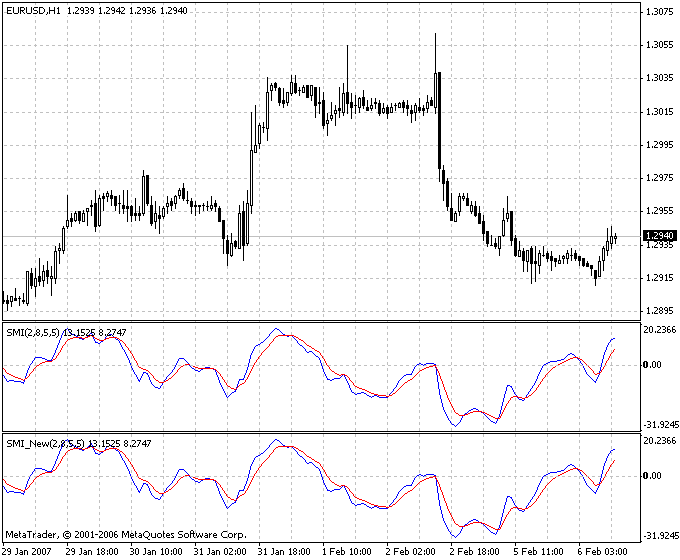Substituting the Function IndicatorCounted()

Now let us analyze the emulation of the function IndicatorCounted(). This function returns the amount of the current chart bars that are unchanged after the last indicator call. Or we can say it in other words. This function returns the amount of the current chart bars that were available in the client terminal on the previous tick. For values to be absolutely identical, we need to subtract one from the resulting amount of bars. So this function can be easily substituted for a static integer variable, which will be initialized by the value of a predetermined variable Bars-1 after a value is received from it. After that the indicator scheme will have the following form:
```//+------------------------------------------------------------------+
//|                                            NewIndicatorPlan1.mqh |
//|                                        https://www.metaquotes.net/ |
//+------------------------------------------------------------------+

//---- INPUT INDICATOR PARAMETERS
extern int period0 = 15;
extern int period1 = 15;
extern int period2 = 15;
//---- indicator buffers
double Ind_Buffer0[];
double Ind_Buffer1[];
double Ind_Buffer2[];
//+------------------------------------------------------------------+
//| Custom indicator initialization function                         |
//+------------------------------------------------------------------+
int init()
{
//---- initialization end
return(0);
}
//+------------------------------------------------------------------+
//| Custom indicator iteration function                              |
//+------------------------------------------------------------------+
int start()
{
//---- Checking whether the bars number is enough for further calculation
if(Bars < period0 + period1 + period2)
return(0);
//---- INDICATOR BUFFERS EMULATION
if(ArraySize(Ind_Buffer0) < Bars)
{
ArraySetAsSeries(Ind_Buffer0, false);
ArraySetAsSeries(Ind_Buffer1, false);
ArraySetAsSeries(Ind_Buffer2, false);
//----
ArrayResize(Ind_Buffer0, Bars);
ArrayResize(Ind_Buffer1, Bars);
ArrayResize(Ind_Buffer2, Bars);
//----
ArraySetAsSeries(Ind_Buffer0, true);
ArraySetAsSeries(Ind_Buffer1, true);
ArraySetAsSeries(Ind_Buffer2, true);
}
//----+ INSERTION OF A STATIC INTEGER MEMORY VARIABLE
static int IndCounted;
//----+ Insertion of variables with a floating point
double Resalt0, Resalt1, Resalt2;
//----+ Insertion of integer variables and getting calculated bars
int limit, MaxBar, bar, counted_bars = IndCounted;
//---- checking for possible errors
if(counted_bars < 0)
return(-1);
//---- the last calculated bar must be recalculated
if(counted_bars > 0)
counted_bars--;
//----+ REMEMBERING THE AMOUNT OF ALL BARS OF THE CHART
IndCounted = Bars - 1;
//---- defining the number of the oldest bar,
//     starting from which new bars will be recalculated
limit = Bars - counted_bars - 1;
//---- defining the number of the oldest bar,
//     starting from which new bars will be recalculated
MaxBar = Bars - 1 - (period0 + period1 + period2);
//---- initialization of zero
if(limit > MaxBar)
{
limit = MaxBar;
for(bar = Bars - 1; bar >= 0; bar--)
{
Ind_Buffer0[bar] = 0.0;
Ind_Buffer1[bar] = 0.0;
Ind_Buffer2[bar] = 0.0;
}
}
//----+ THE FIRST CYCLE OF INDICATOR CALCULATION
for(bar = limit; bar >= 0; bar--)
{
// Here code of the variable Resalt1 calculation
// based on the external variable period1
Ind_Buffer1[bar] = Resalt1;
}
//----+ THE SECOND CYCLE OF INDICATOR CALCULATION
for(bar = limit; bar >= 0; bar--)
{
// Here code of the variable Resalt2 calculation
// based on the values of the buffer Ind_Buffer1[] and external variable period2
Ind_Buffer2[bar] = Resalt2;
}
//----+ THE MAIN CYCLE OF INDICATOR CALCULATION
for(bar = limit; bar >= 0; bar--)
{
// Here code of the variable Resalt0 calculation
// based on the values of the buffer Ind_Buffer2[] and external variable period0
Ind_Buffer0[bar] = Resalt0;
}
return(0);
}
//+------------------------------------------------------------------+```

Further Transformation of the Indicator Code and the Final Scheme of Its Structure

Of course, we could simply transfer the indicator code in parts into an EA code, supposing we need this indicator in an Expert Advisor for the operation on the current chart and only once! If the indicator is used twice, we could simply change in the second case names of all indicator variables and add the code once again. But in this case the Expert Advisor will become more complicated.

The task of processing data on other timeframes is also solved easily. Substitute predetermined variables of Bars type for timeseries of the type
`iBars(string symbol,  int timeframe);`

NULL - for string symbol;, 0 (in timeseries) - for int timeframe;, Close[bar] - for

`iClose(string symbol, int timeframe, bar);`

and so on.

Now let us analyze the indicator lines:

```//---- checking for possible errors
if(counted_bars < 0)
return(-1);
//---- the last calculated bar must be recalculated
if(counted_bars > 0)
counted_bars--;```
As for the offered substitution of the function IndicatorCounted() in our variant of the indicator structure, the indicator counted_bars will never be less than zero. So the lines:

```//---- checking for possible errors
if(counted_bars < 0)
return(-1);```
in the Expert Advisor code can be omitted. With the next two lines:

```//---- the last calculated bar must be recalculated
if(counted_bars > 0)
counted_bars--;```

it is the same. They should be deleted, while they just slow down the EA work by unnecessary recalculations of the firs bar and in the EA operation this check is absolutely useless. After that the final code for transferring into an EA has the following form:

```//+------------------------------------------------------------------+
//|                                            NewIndicatorPlan2.mqh |
//|                                        https://www.metaquotes.net/ |
//+------------------------------------------------------------------+

//---- INPUT INDICATOR PARAMETERS
extern int period0 = 15;
extern int period1 = 15;
extern int period2 = 15;
//---- indicator buffers
double Ind_Buffer0[];
double Ind_Buffer1[];
double Ind_Buffer2[];
//---- DECLARING VARIABLES FOR CHOOSING A CHART
string symbol; int timeframe;
//+------------------------------------------------------------------+
//| Custom indicator initialization function                         |
//+------------------------------------------------------------------+
int init()
{
//---- CHOOSING A CHART FRO INDICATOR CALCULATION
symbol = Symbol();//INITIALIZATION OF THE VARIABLE symbol;
timeframe =240;//INITIALIZATION OF THE VARIABLE timeframe;
//---- end of initialization
return(0);
}
//+------------------------------------------------------------------+
//| Custom indicator iteration function                              |
//+------------------------------------------------------------------+
int start()
{
// GETTING THE AMOUNT OF ALL BARS OF THE CHART
int IBARS = iBars(symbol, timeframe);
//---- Checking whether the bars number is enough for further calculation
if(IBARS < period0 + period1 + period2)
return(0);
// INDICATOR BUFFERS EMULATION
if(ArraySize(Ind_Buffer0) < IBARS)
{
ArraySetAsSeries(Ind_Buffer0, false);
ArraySetAsSeries(Ind_Buffer1, false);
ArraySetAsSeries(Ind_Buffer2, false);
//----
ArrayResize(Ind_Buffer0, IBARS);
ArrayResize(Ind_Buffer1, IBARS);
ArrayResize(Ind_Buffer2, IBARS);
//----
ArraySetAsSeries(Ind_Buffer0, true);
ArraySetAsSeries(Ind_Buffer1, true);
ArraySetAsSeries(Ind_Buffer2, true);
}
// INSERTION OF A STATIC INTEGER MEMORY VARIABLE
static int IndCounted;
//----+ Insertion of variables with a floating point
double Resalt0, Resalt1, Resalt2;
//----+ Insertion of integer variables and GETTING ALREADY CALCULATED BARS
int limit, MaxBar, bar, counted_bars = IndCounted;
//----+ REMEMBERING THE AMOUNT OF ALL BARS OF THE CHART
IndCounted = IBARS - 1;
//---- defining the number of the oldest bar,
//     starting from which new bars will be recalculated
limit = IBARS - counted_bars - 1;
//---- defining the number of the oldest bar,
//     starting from which new bars will be recalculated
MaxBar = IBARS - 1 - (period0 + period1 + period2);
//---- initialization of zero
if(limit > MaxBar)
{
limit = MaxBar;
for(bar = IBARS - 1; bar >= 0; bar--)
{
Ind_Buffer0[bar] = 0.0;
Ind_Buffer1[bar] = 0.0;
Ind_Buffer2[bar] = 0.0;
}
}
//----+ THE FIRST CYCLE OF INDICATOR CALCULATION
for(bar = limit; bar >= 0; bar--)
{
// Here code of the variable Resalt1 calculation based on the external
// variable period1
Ind_Buffer1[bar] = Resalt1;
}
//----+ THE SECOND CYCLE OF INDICATOR CALCULATION
for(bar = limit; bar >= 0; bar--)
{
// Here code of the variable Resalt2 calculation
// based on the values of the buffer Ind_Buffer1[] and the external variable period2
Ind_Buffer2[bar] = Resalt2;
}
//----+ THE MAIN CYCLE OF INDICATOR CALCULATION
for(bar = limit; bar >= 0; bar--)
{
// Here code of the variable Resalt0 calculation
// based on the values of the buffer Ind_Buffer2[] and the external variable period0
Ind_Buffer0[bar] = Resalt0;
}
return(0);
}
//+------------------------------------------------------------------+```
Here we should take into account one moment. There are indicators, which at the multiple recalculation of the zero bar, on the first bar at the beginning of the calculation cycles remember the values of some variables for returning the code into its initial state (Article). In an Expert Advisor after deleting the last two lines this remembering should take place on the zero bar, and not on the first one. Usually such indicators contain at the beginning of calculation cycles the following code fragments:
```// Saving the variables values
if(bar == 1)
if(((limit == 1) && (time == Time)) || (limit > 1))
{
time = Time;
// These variables are not interesting for us
PRICE = price;
TREND = trend;
RESALT = Resalt;
}
//+------------------------------------------------------------------+```

This fragment should be amended for saving variable values not on the first, but on the zero bar. All '1' should be substituted by '0', '2' - by '1'. And if the code is intended for operation not on the current chart, reference to a timeseries array should also be changed

`time = Time;`

into

`time = iTime(symbol, timeframe, 1);`

As a result we have the following changed fragment:

```// Saving variables values
if(bar == 0)
if(((limit == 0) && (time == iTime(symbol, timeframe, 1))) || (limit > 0))
{
time = iTime(symbol, timeframe, 1);
PRICE = price;
TREND = trend;
RESALT = Resalt;
}
//+------------------------------------------------------------------+```

An indicator code can also contain the functions of smoothing like XXXSeries(), developed by myself. For using in an EA code the fragments with such functions, these functions should be substituted for their EA analogues.

### Conclusion

Translated from Russian by MetaQuotes Software Corp.
Original article: https://www.mql5.com/ru/articles/1456

Attached files |
IndicatorPlan1.mq4 (6.05 KB)
SMI.MQ4 (4.87 KB)
SMI_New.MQ4 (5.93 KB)

#### Other articles by this author

Last comments | Go to discussion (4)[Deleted] | 12 Apr 2012 at 01:28

Hi,

Pardon my question as I'm still new to MQL4 .

Why all this pain of conversion from indicator to EA when we can use icustom to "insert" the indicator into EA?

What are the disadvantages of doing this?| 25 Nov 2015 at 22:34

Thank you Nikolay for your very good article and the information disclosed. I myself have problems with an indicator that I have written which works very well on the chart. However, it returns totally wrong values when called from an EA. I hope that I will be able to solve the problem after digesting your article.

However, I am a bit confused regarding the solutions that you suggest. There are two examples of solutions: IndicatorPlan1 and IndicatorPlan2. What is the difference? Which one is the final solutions. Also, I am not sure I understand what you suggest. Should one paste the indicator code inside the EA? This would make the EA very complex and cumbersome. I would rather be able to call the custom indicator from inside the EA using "iCustom". Can you please elaborate on that and on how to use it in combination with an EA?

Thanks again

Omar| 2 Aug 2018 at 18:46

hi

Preparing the indicator and EA both are different and the both of the files are in different  loacation

Now while runing the indicator call the EA CODE?

my question is how can we call the EA into the indicator ?

How can do the code EA code is called into the Indicator ?| 8 Jan 2019 at 05:02
The EA that was made doesnt work. So whats the point, if you have made a post about conversion from an indicator to EA and its not even successful?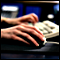Problems of Technical Analysis Revisited
At present, technical analysis along with the fundamental one is the most important method to analyze stock market. Being one of the predicting methods of stock market pricing dynamics, the technical analysis has a large amount of disadvantages that cast some doubt on its practical applicability.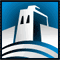MetaTrader 4 Working under Antiviruses and Firewalls
The most of traders use special programs to protect their PCs. Unfortunately, these programs don't only protect computers against intrusions, viruses and Trojans, but also consume a significant amount of resources. This relates to network traffic, first of all, which is wholly controlled by various intelligent antiviruses and firewalls. The reason for writing this article was that traders complained of slowed MetaTrader 4 Client Terminal when working with Outpost Firewall. We decided to make our own research using Kaspersky Antivirus 6.0 and Outpost Firewall Pro 4.0.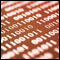Transferring an Indicator Code into an Expert Advisor Code. General Structural Schemes of an Expert Advisor and Indicator Functions
This article dwells on the ways of transferring an indicator code into an Expert Advisor Code and on writing Expert Advisors with no calling to custom indicators, and with the whole program code for the calculation of necessary indicator values inside the Expert Advisor. This article gives a general scheme of Expert Advisor changing and the idea of building an indicator function based on a custom indicator. The article is intended for readers, already having experience of programming in MQL4 language.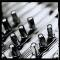Indicator Alternative Ichimoku – Setup, Examples of Usage
How to set up Alternative Ichimoku correctly? Read the description of parameters setting up. The article will help you understand the methods of setting up parameters not only of the indicator Ichimoku. Certainly you will also better understand how to set up the standard Ichimoku Kinko Hyo.